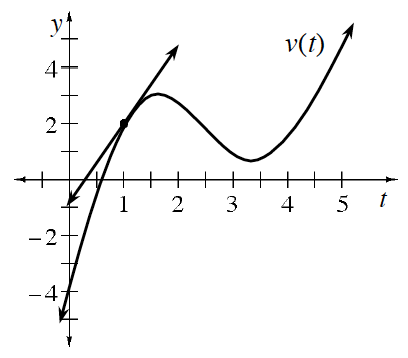### Home > APCALC > Chapter 6 > Lesson 6.4.1 > Problem6-123

6-123.

Trace the distance-time graph and the tangent at $t = 1$ second on your paper. Distance $d$ is measured in feet.

1. Estimate the velocity at $t = 1$ s.

Estimate the slope of the tangent.

2. Locate another time on the graph where the object has the same velocity as $t = 1$. Use you graph to show how you know the velocities are equal.

Sketch various tangent lines to the graph of $y = d(t)$. Keep sketching until you find one with the same slope as the tangent at $t = 1$.

Parallel lines have the same slope.